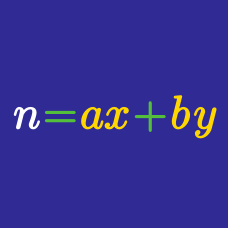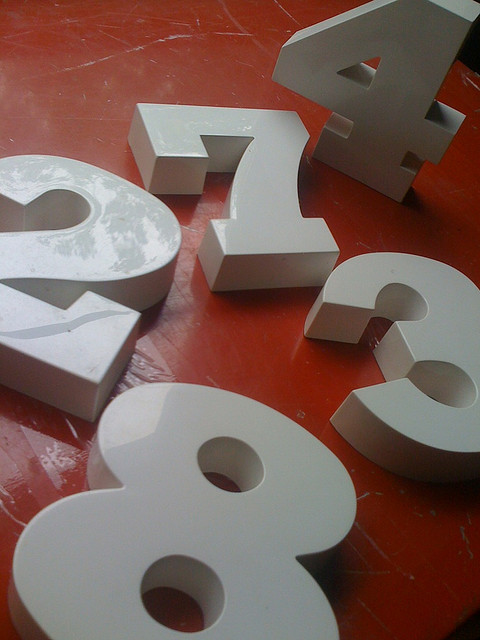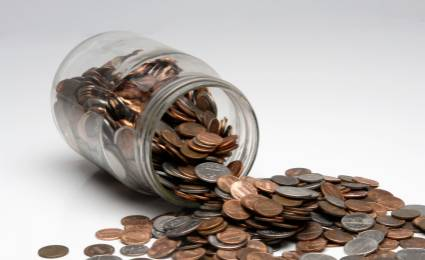Number Theory

Linear Diophantine Equations: Level 2 ChallengesWhat is the positive four-digit integer for which:

• the first (in other words, the left-most) digit is one-third the second digit,
• the third digit is the sum of the first and second digits,
• and the last digit is three times the second digit?

Find the two digit positive integer that is equal to:

• one more than eight times the sum of its digits

AND

• two more than the product of 13 and the positive difference between its digits.

$\large{\begin{array}{ccccccc} && & & \color{#3D99F6}X& \color{#3D99F6}X & \color{#3D99F6}X&\color{#3D99F6}X\\ && & & \color{#20A900}Y& \color{#20A900}Y & \color{#20A900}Y&\color{#20A900}Y\\ +&& & & \color{#D61F06}Z& \color{#D61F06}Z & \color{#D61F06}Z&\color{#D61F06}Z\\ \hline & & & \color{#20A900}Y& \color{#3D99F6}X& \color{#3D99F6}X & \color{#3D99F6}X&\color{#D61F06}Z\\ \hline \end{array}}$

If $\color{#3D99F6}X$, $\color{#20A900}Y$ and $\color{#D61F06}Z$ are distinct digits in the sum above, then find $\color{#D61F06}Z$.If you have infinite pennies ($0.01), nickels ($0.05), and dimes ($0.10), in how many different ways can you make change for$1.00?

Hint: Don't try to list all of the possibilities!

Gummy bears come in packs of 6 and 9. Is there a combination of these packs which gives a total of exactly 100 gummy bears?

×